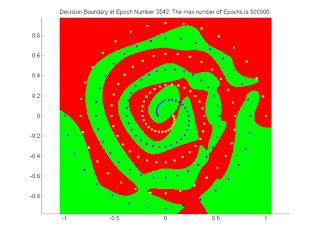## Thursday, November 19, 2015

### MLP Neural Network with Backpropagation [MATLAB Code]

This is an implementation for Multilayer Perceptron (MLP) Feed Forward Fully Connected Neural Network with a Sigmoid activation function. The training is done using the Backpropagation algorithm with options for Resilient Gradient Descent, Momentum Backpropagation, and Learning Rate Decrease. The training stops when the Mean Square Error (MSE) reaches zero or a predefined maximum number of epochs is reached.

Four example data for training and testing are included with the project. They are generated by SharkTime Sharky Neural Network.

http://www.mathworks.com/matlabcentral/fileexchange/54076-mlp-neural-network-with-backpropagation

2- Network architecture & Training Parameters:
The code configuration parameters are as follows:

1- Numbers of hidden layers and neurons per hidden layer. It’s represented by the variable nbrOfNeuronsInEachHiddenLayer. To have a neural network with 3 hidden layers with number of neurons 4, 10, and 5 respectively; that variable is set to [4 10 5].
2- Number of output layer nits. Usually the number of output units is equal to the number of classes, but it still can be less (≤ log2(nbrOfClasses)). It’s represented by the variable nbrOfOutUnits. The number of input layer units is obtained from the training samples dimension.
3- The selection if the sigmoid activation function is unipolar or polar. It’s represented by the variable unipolarBipolarSelector.
4- The learning rate η.
5- The maximum number of epochs at which the training stops unless MSE reaches zero. It’s represented by the variable nbrOfEpochs_max.
6- Option to enable or disable Momentum Backpropagation. It’s represented by the variable enable_learningRate_momentum.
7- The Momentum Backpropagation rateα. It’s represented by the variable momentum_alpha.
8- Option to enable or disable Resilient Gradient Descent. It’s represented by the variable enable_resilient_gradient_descent.
9- The Resilient Gradient Descent parametersη+η-Δmin, Δmax, represented by the variables learningRate_plus, learningRate_negative, deltas_min, and deltas_max.
10- Option to enable or disable Learning Rate Decrease. It’s represented by the variable enable_decrease_learningRate.
11- The Learning Rate Decrease parameters:and. It’s represented by the variables learningRate_decreaseValue and min_learningRate.

The code also contains a parameter for drawing the decision boundary separating the classes and the MSE curve. The number of epochs after which a figure is drawn and saved on the machine is specified. The figures are saved in a folder named Results besides the m files. This parameter is represented by the variable draw_each_nbrOfEpochs. The variable dataFileName takes the Sharky input points file name as string.

3- Results:
In all of the following four test cases, MCCR=1. The stop condition is that MSE reaches zero in all the cases. A unipolar sigmoid function is chosen.

A) Linear Points Case:
Figure 1. Network: 2-4-2 , Unipolar Sigmoid Activation, No Options, η=0.15.

B) Circle Points Case:

Figure2. Network: 2-10-2 , Unipolar Sigmoid Activation, Resilient Gradient Descent, η+=1.2, η-=0.5, Δmin=10^-6, Δmax=50.

C) Wave Points Case:
Figure 3. Network: 2-10-10-2 , Unipolar Sigmoid Activation, Resilient Gradient Descent, η+=1.2, η-=0.5, Δmin=10^-6, Δmax=50.

D) Spiral Points Case:

Figure 4. Network: 2-10-10-2 , Unipolar Sigmoid Activation, Resilient Gradient Descent, η+=1.2, η-=0.5, Δmin=10^-6, Δmax=50.

The following image shows a magnification for the decision boundary with better resolution.Figure 5. Magnified decision boundary with better resolution for Spiral points case.

The following video shows the solving of the Two Spirals problem.

Video 1. Solving the Two Spirals Problem.